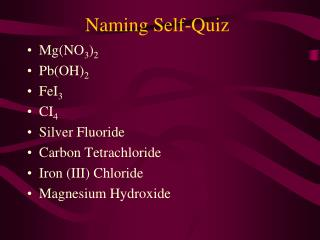DownloadDownload PresentationNaming Self-Quiz

# Naming Self-Quiz

Télécharger la présentation## Naming Self-Quiz

- - - - - - - - - - - - - - - - - - - - - - - - - - - E N D - - - - - - - - - - - - - - - - - - - - - - - - - - -
##### Presentation Transcript

1. Naming Self-Quiz Mg(NO3)2 Pb(OH)2 FeI3 CI4 Silver Fluoride Carbon Tetrachloride Iron (III) Chloride Magnesium Hydroxide

2. Developing Chemical Equations

3. Chemical Equations A chemical change involves conversion of substances into other substances Reactants  Products Reactants: pure substances that undergo a chemical change Products: pure substance that is formed in a chemical change (different properties than reactants)

4. Writing Chemical Equations 3 ways to represent chemical reactions: Word Equations Skeleton Equations Balanced Chemical Equations

5. Word Equations Describes a chemical reaction stating the reactants and products Reactant 1 + Reactant 2  Product 1 + Product 2 Example: hydrogen + oxygen  water

6. Skeleton Equation Replaces words with chemical formulas to show the chemical composition of the substances Example H2+ O2  H2O

7. States The state of any substance in a chemical equation can be described using one of the following symbols:

8. States Example H2(g)+ O2(g)  H2O(l)

9. Law of Conservation of Mass In any given chemical reaction, the total mass of the products is always the same as the total mass of the reactants

10. Examples 4g hydrogen + 32 grams oxygen  36 grams water 103g potassium oxide + 9g water  112g potassium hydroxide 1g carbon + 5.34g sulfur  6.34g carbon disulfide

11. Balanced Chemical Equations Shows the units of reactants and products Demonstrates the law of conservation of mass  same number of atoms of each element to appear on both sides of the equation

12. Coefficient : # placed in front of a chemical formula in a balanced chemical equation Example 2H2 + O2 2H2O 4 hydrogen + 2 oxygen  4 hydrogen, 2 oxygen

13. When balancing an equation, you CANNOT add subscripts Subscripts change the identity of the substance 2H2O = 2 water H2O2 = hydrogen peroxide

14. Examples Word Equation Aluminum + Oxygen  Aluminum Oxide Skeleton Al(s) + O2(g) Al2O3(s) Balanced 4Al(s) + 3O2(g)2Al2O3(s)

15. Examples Word Equation Aluminum oxide + Calcium Calcium Oxide + Aluminum Skeleton Al2O3(s) + Ca(s) CaO (s) + Al(s) Balanced Al2O3(s) + 3Ca(s)3CaO(s) + 2Al(s)

16. Examples Word Equation Calcium Chloride + Sodium Sulfate  Calcium Sulfate + Sodium Chloride Skeleton CaCl2 + Na2SO4 CaSO4 + NaCl Balanced CaCl2 + Na2SO4 CaSO4 + 2NaCl

17. Examples Word Equation Carbon Tetrahydride + Oxygen Gas  Carbon Dioxide + Water Skeleton CH4 + O2 CO2 + H2O Balanced CH4 + 2O2 CO2 + 2H2O

18. WORK Pg. 236 #3-7